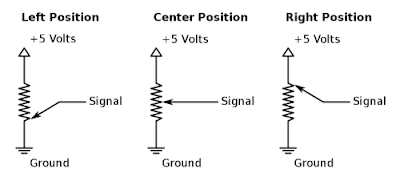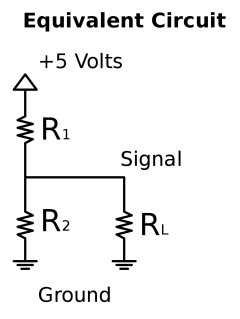## Tuesday, September 16, 2008

### Analog to Digital Conversion

This tutorial covers some of the basics for connecting an analog sensor to a PIC microcontroller.

In this case we will be using a potentiometer as our sensor, but accelerometers, gyroscopes, temperature sensors, and most other analog sensors will work in a similar way.As the potentiometer is rotated approximately 315 degrees from left to right the signal voltage will vary between 0 Volts and 5Volts, In this example the output signal will be connected to pin AN0 on the PIC microcontroller. The equivalent circuit shows how the potentiometer can be represented as a voltage divider.By making the assumption the RL is very large, the output signal voltage is a function of the input voltage and the position of the potentiometer knob.
{tex}$V_{out}=V_{in}\frac{R_{2}}{R_{1}+R_{2}}${/tex}

In practice your input voltage is almost never 5 Volts, and your sensor is never absolutely linear, so probably the best thing to do is to calibrate in software or use a high precision voltage reference. The voltage reference setup is a little more complicated so it will be left for the reader, however here is a series voltage reference from Texas Instruments that would probably work well.
2.5V 100ppm/Degrees C, 50uA in SOT23-3 Series (Bandgap) Voltage Reference
This App Note from Maxim has more information about choosing a voltage reference if you are really interested.
Series or Shunt Voltage Reference?
So ignoring the problems for now, we can determine the sensitivity of the potentiometer.

{tex}$Sensitivity=\frac{V_{in}}{\theta_{max}-\theta_{min}}$=$\frac{5V*1000}{315\textdegree}\approx15.87mV/\textdegree${/tex}
The range of motion of the potentiometer is estimated to be 315 degrees and the full voltage range is considered valid.

{tex}$Resolution=\frac{V_{in}}{2^{n}}=\frac{5V}{1024bits}\approx4.88mV/bit${/tex}
On a PIC 18F4550 the A/D Converter is 10 bits, on the 18F4553 it is 12 bits, these values are substituted in for n.

With these values, the number of degrees of rotation can be determined from the value returned in software by ReadADC()
Keep in mind that you will not get 12 bits of accurate data without some serious work. If you really need 12 bits then you should read the following PDF by Bonnie Baker from Microchip.
Techniques that Reduce System Noise in ADC CircuitsSo again skipping the complicated bits, connect the sensor to the PIC microcontroller. In this case we are using Create USB Development boards, but there are many other choices out there.

{tex}$\theta=ADC_{in}*Resolution/Sensitivity\approx ADC_{in}*.3077${/tex}
Theta will be considered to be a positive angle when rotated clockwise from the center position, and a negative angle when rotated to the left.

Using the Microchip USB Framework here is the code fragments necessary to get things working.
the variable x listed in the code below is assumed to be a global float but if you need more speed and less accuracy you can change it.

void UserInit(void)
{
// set AN0 to input
TRISAbits.TRISA0=INPUT_PIN;

// etc...
}

void ProcessIO(void)
{
// if((usb_device_state < CONFIGURED_STATE)||(UCONbits.SUSPND==1)) return;

if (lastcount != count) {
Delay10TCYx( 5 ); // Delay for 50TCY